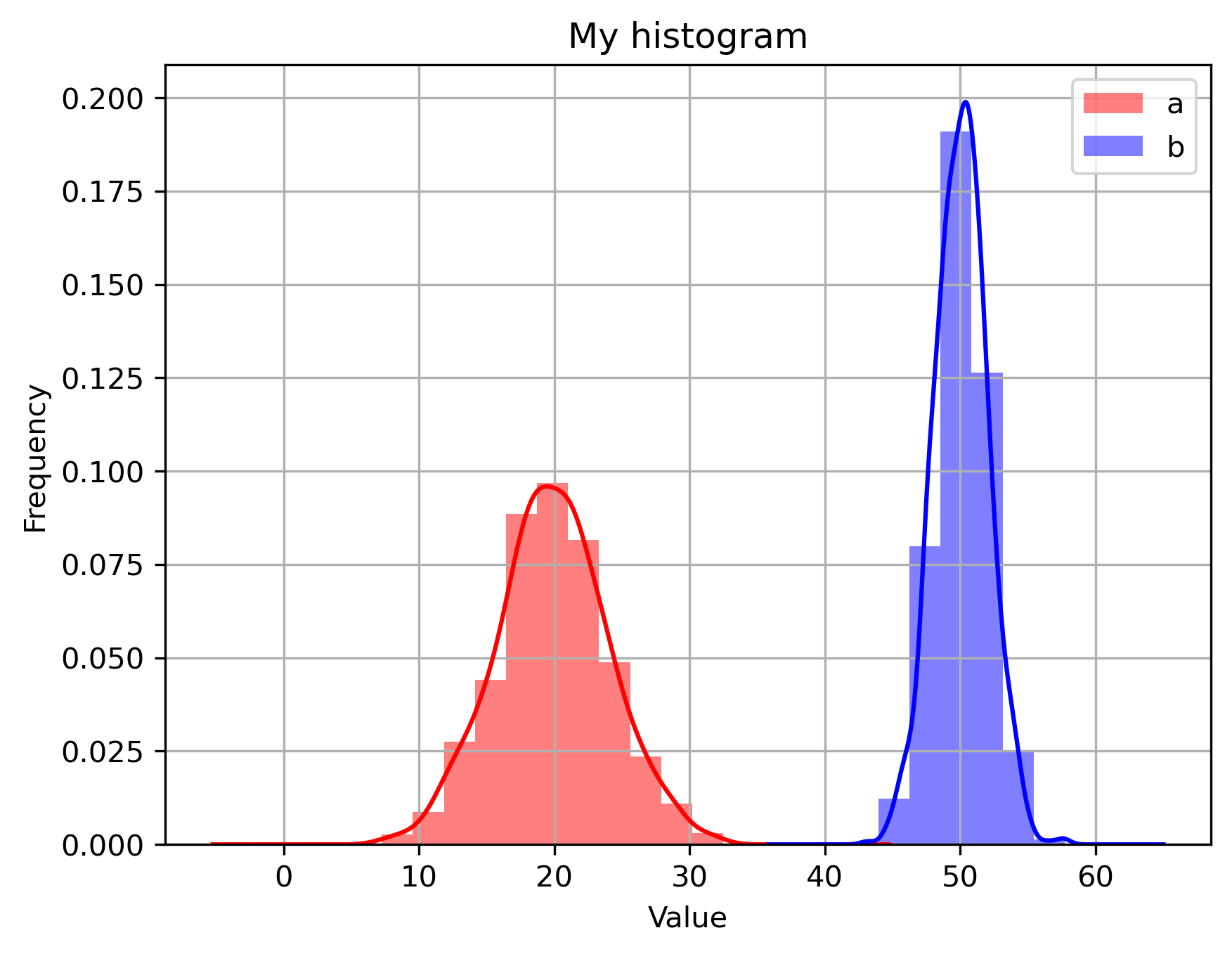# Advanced 2D plots with Matplotlib in Python (codes included)

Codes for plotting advanced 2D plots using matplotlib library in Python. Includes simple 2D plot, error bars, bar graphs, histograms, multiple plots, etc

## Simple 2D plots

Let us make some fake data using the random module from the numpy library and then plot it using matplotlib.

import numpy as np
import matplotlib.pyplot as plt

plt.style.use('seaborn')

# make fake data
x_orig = np.linspace(0, 4, 20) # points between 0 and 4
noise = 0.025*np.random.normal(size=len(x_orig)) # random numbers
y_orig = np.exp(-x_orig) + noise # data is theory plus noise

# create theoretical curve to compare with "data"
x_pred = np.linspace(min(x_orig),max(x_orig), 200) # use more values to get smooth curve
y_pred = np.exp(-x_pred)

# setup the plots: both points and smooth curve
plt.plot(x_orig, y_orig, 'bo', label='data', lw=3) # points
plt.plot(x_pred, y_pred, color='green', label='pred', lw=3) # line
# plt.grid() #can use this if the style is not imported
plt.legend()
plt.xlabel('x')
plt.ylabel('Original vs Predicted')
plt.title("Results")

plt.savefig('simple_plot_non_log.png',dpi=300,bbox_inches='tight')
plt.close('all')


## Similar posts

#### How to plot the boundaries of the states on the basemap of the usa

We used the style seaborn. Alternatively, many other styles can be used like classic, ggplot, etc. The noise is generated by taking samples from the gaussian distribution. The np.linspace function generates the equally distributed 200 points between the min and max of x_orig. For saving the plot, the argument bbox_inches crop the white spaces in the figure and dpi set the print resolution of the image. Finally, we close the plot using plt.close('all'). This comes in handy when you use the for loop to save many figures iteratively.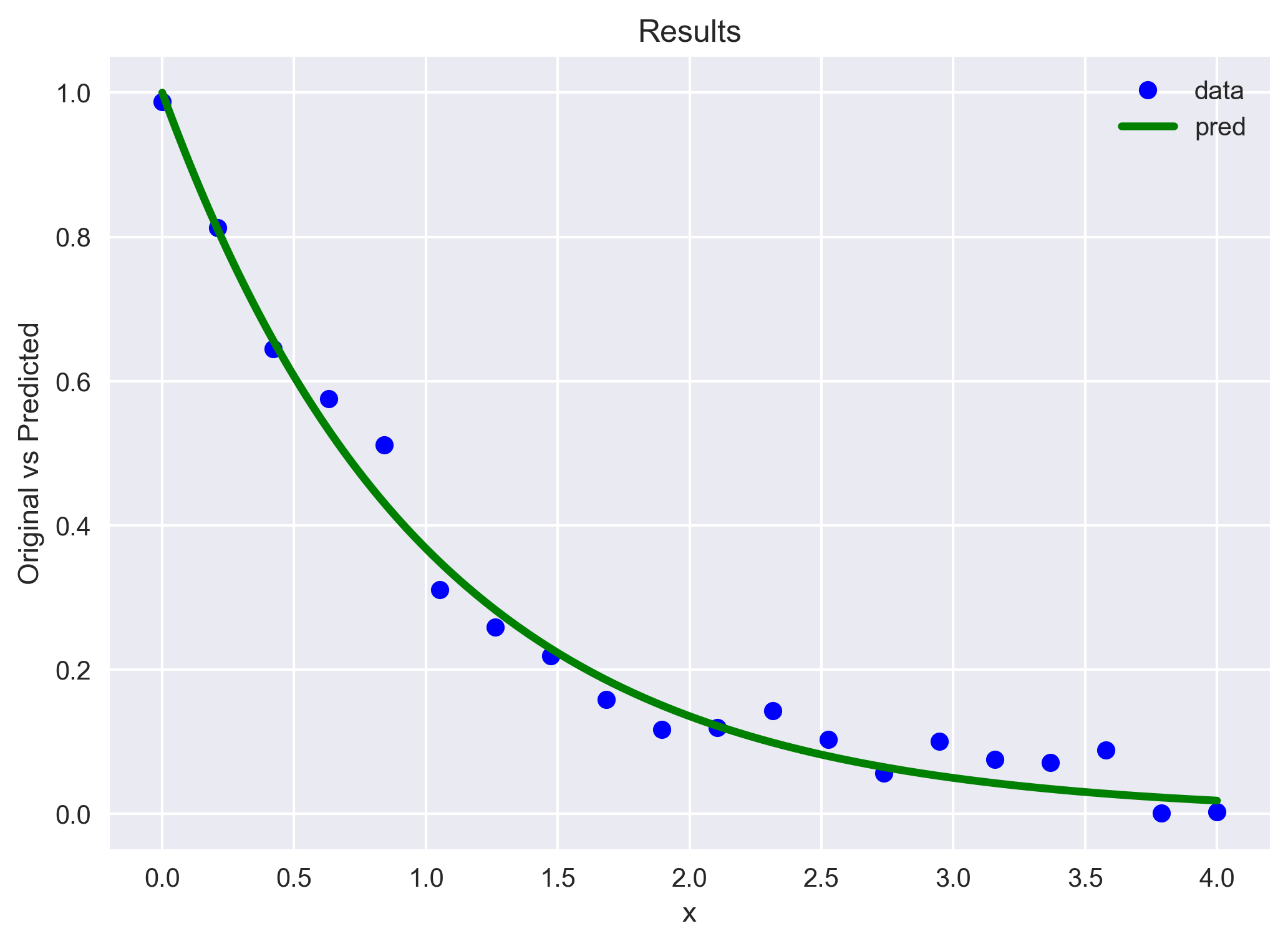Now, we linearize this plot using the log of the y axis (plt.yscale)

# setup the plots: both points and smooth curve
fig= plt.figure()
plt.plot(x_orig, y_orig, 'bo', label='data', lw=3) # points
plt.plot(x_pred, y_pred, color='green', label='pred', lw=3) # line
# plt.grid() #can use this if the style is not imported
plt.legend()
plt.xlabel('x')
plt.ylabel('Original vs Predicted in log')
plt.title("Results")
plt.yscale('log') # make the y axis (ordinate) log; that is, log-linear

plt.savefig('simple_plot.png',dpi=300,bbox_inches='tight')
plt.close('all') # its a good practice to close all the figures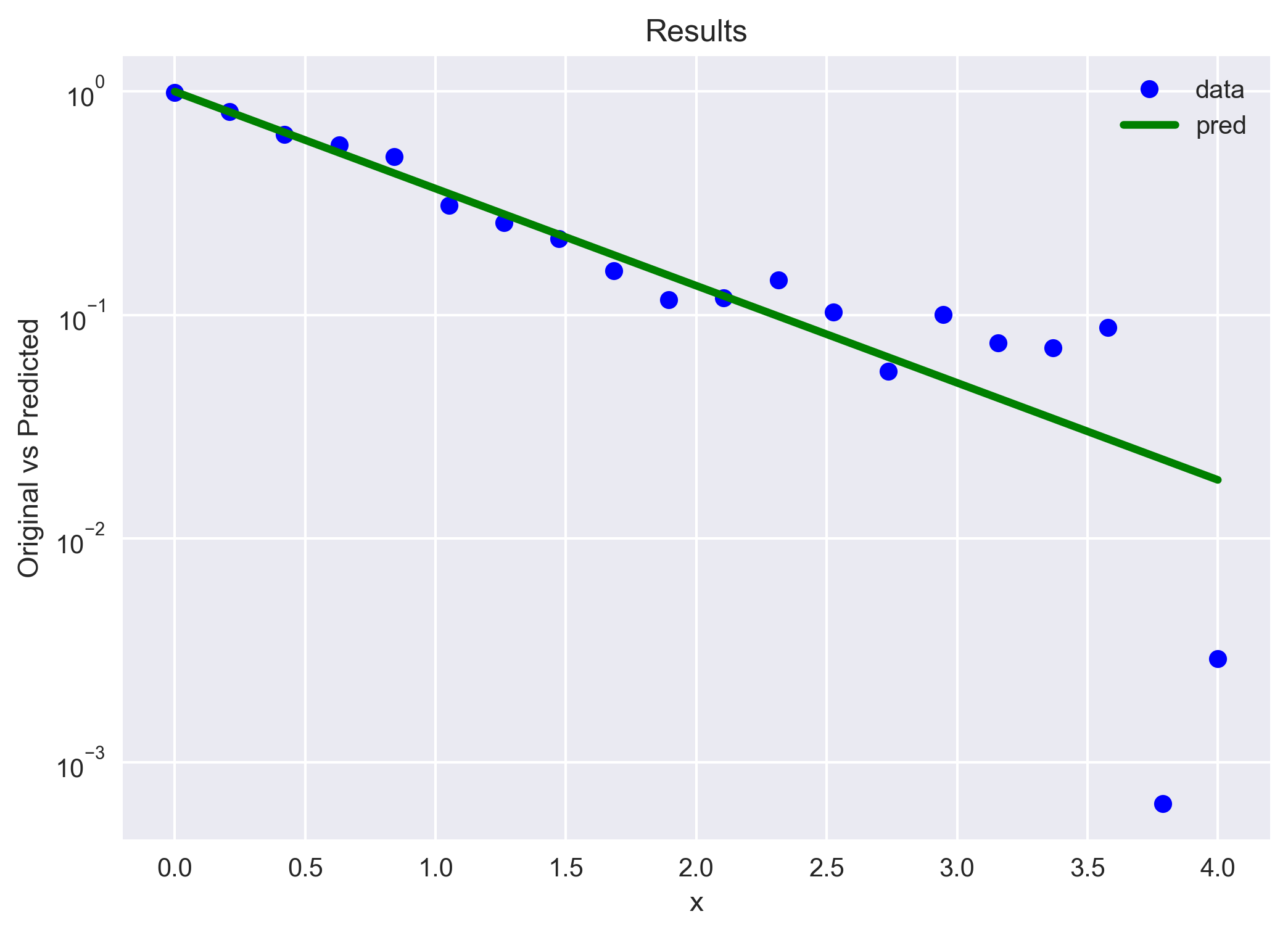## Error bars on the data

Sometimes we need to show the error bars on the measurements as a graphical representation of the variability of data or to indicate the error or uncertainty in a reported measurement.

The error bars give a general idea of how precise a measurement is, or conversely, how far from the reported value the true (error free) value might be.

import numpy as np
import matplotlib.pyplot as plt

# make fake data
x_orig = np.linspace(0, 4, 20) # points between 0 and 4
noise = 0.025*np.random.normal(size=len(x_orig)) # random numbers
y_orig = np.exp(-x_orig) + noise # data is theory plus noise

# including the error bar at each point (10% of the originals)
x_err = x_orig*0.1
y_err = y_orig*0.1

# add to plot the data as (x,y) with error bars
plt.errorbar(x_orig, y_orig, yerr = y_err, xerr = x_err, lw=1,
ecolor='g', fmt='o-', capthick=2, label='data')

plt.title('Experiment Results')
plt.ylabel('ylabel')
plt.xlabel('xlabel')
plt.legend()
plt.grid()

plt.savefig('error_bars.png',dpi=300,bbox_inches='tight')
plt.close('all')



For this example, we arbitrarily took the error bars at each point to be 10% of the original value. We showed the errors in both x and y directions.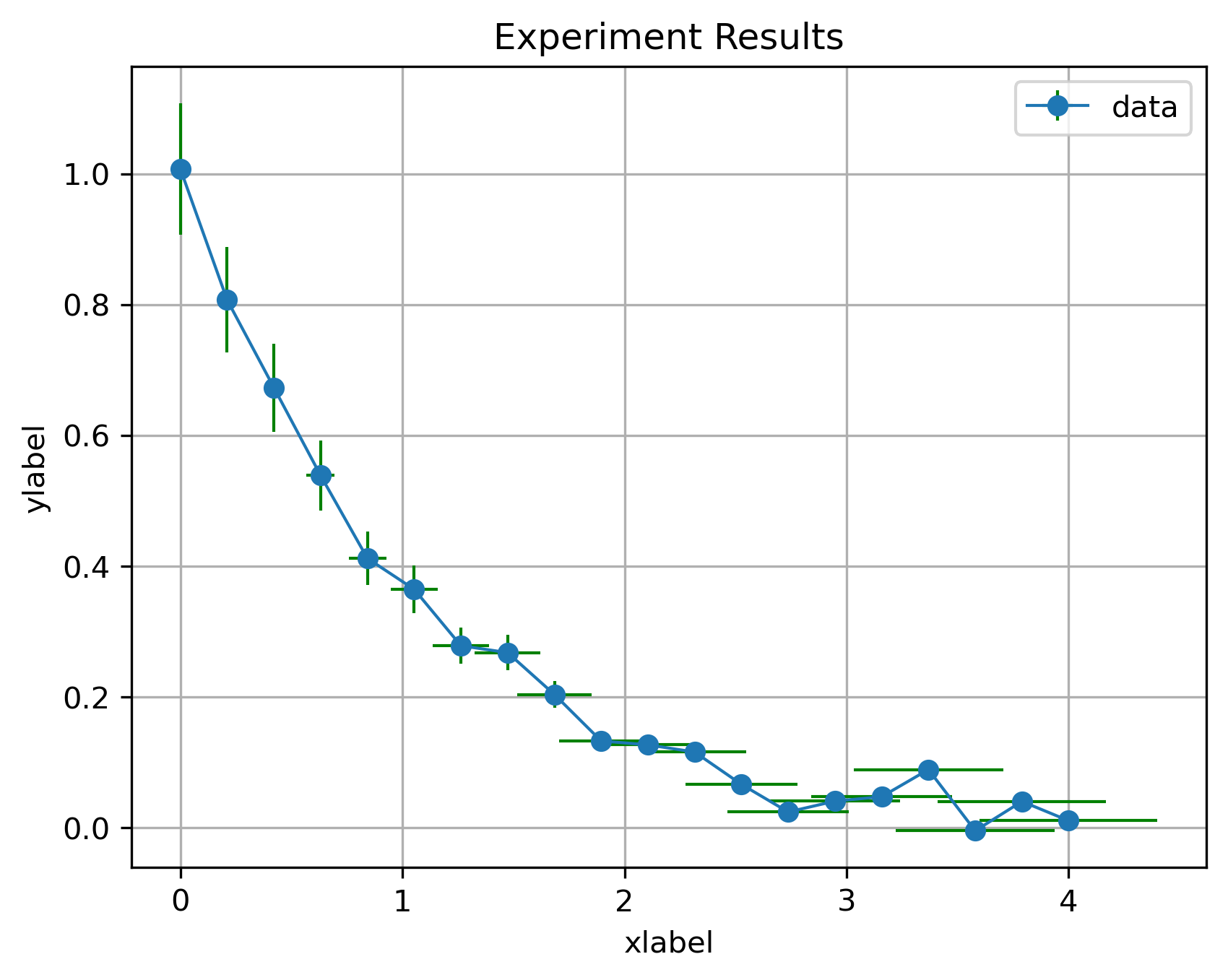## Bar plot

Bar charts are best suited for categorical data. It answers the question of “how many”.

It is important to keep in mind that when the number of categories in your dataset is huge then bar plot may not be the best way to visualize for your data.

### Simple

import matplotlib.pyplot as plt
import numpy as np

## Parameters
opacity=0.5

fig, ax = plt.subplots()

langs = ['Sub-1', 'Sub-2', 'Sub-3', 'Sub-4', 'Sub-5']
students = [23,17,35,29,12]
ax.bar(langs,students, color='orange', alpha=opacity)

plt.savefig('bar_plots.png',dpi=300,bbox_inches='tight')
plt.close('all')


### More than one bar

import matplotlib.pyplot as plt
import numpy as np

## Parameters
bar_width = 0.25
opacity=0.5

data = [[30, 25, 50, 20],
[40, 23, 51, 17],
[35, 22, 45, 19]]
X = np.arange(4)

fig, ax = plt.subplots()
ax.bar(X, data, color = 'b', width = 0.22, label='A')
ax.bar(X + bar_width, data, color = 'g', width = 0.22, label='B')
ax.bar(X + 2*bar_width, data, color = 'r', width = 0.22, label='C')
plt.legend()
plt.xticks(X + bar_width,X)
plt.savefig('bar_plots2.png',dpi=300,bbox_inches='tight')
plt.close('all')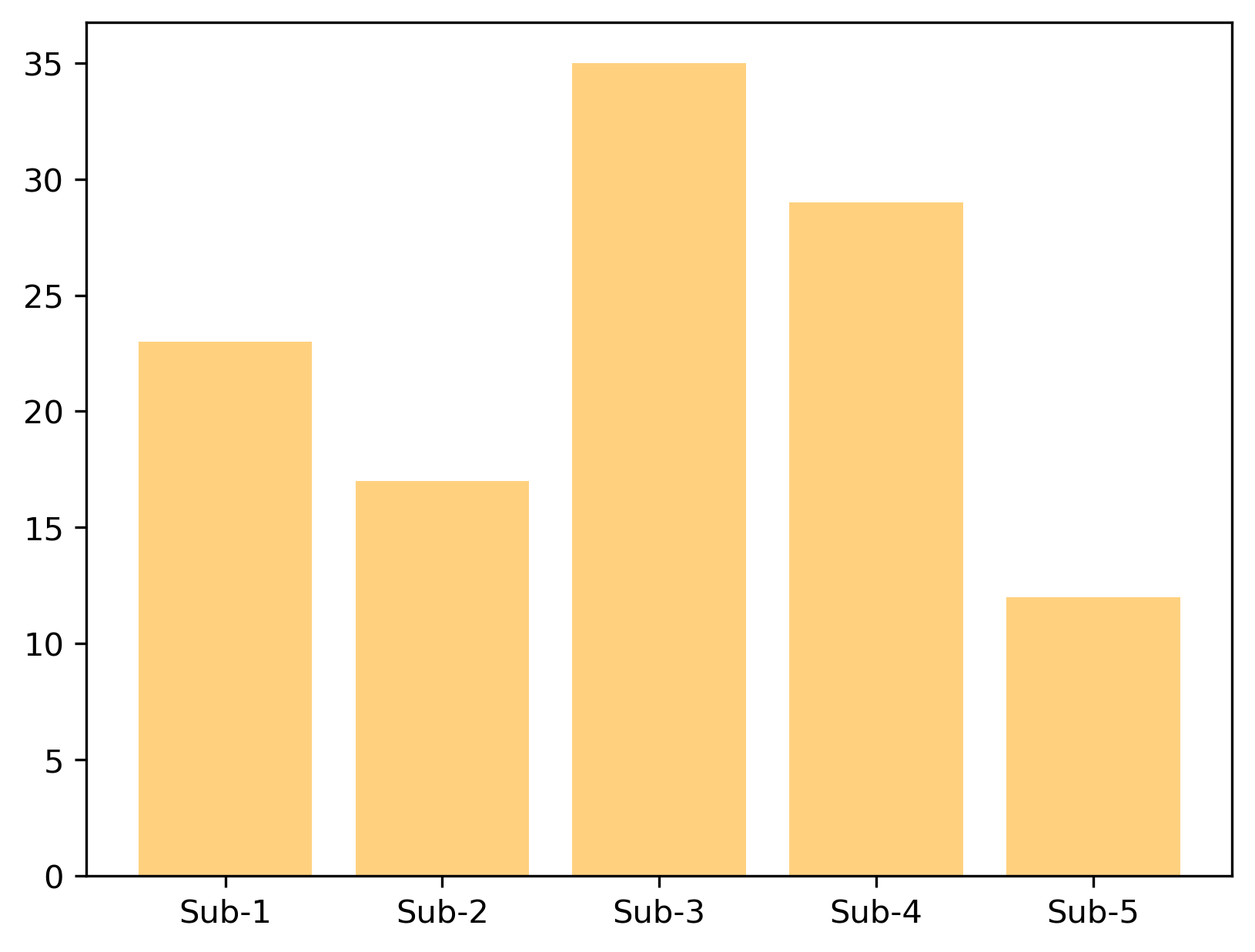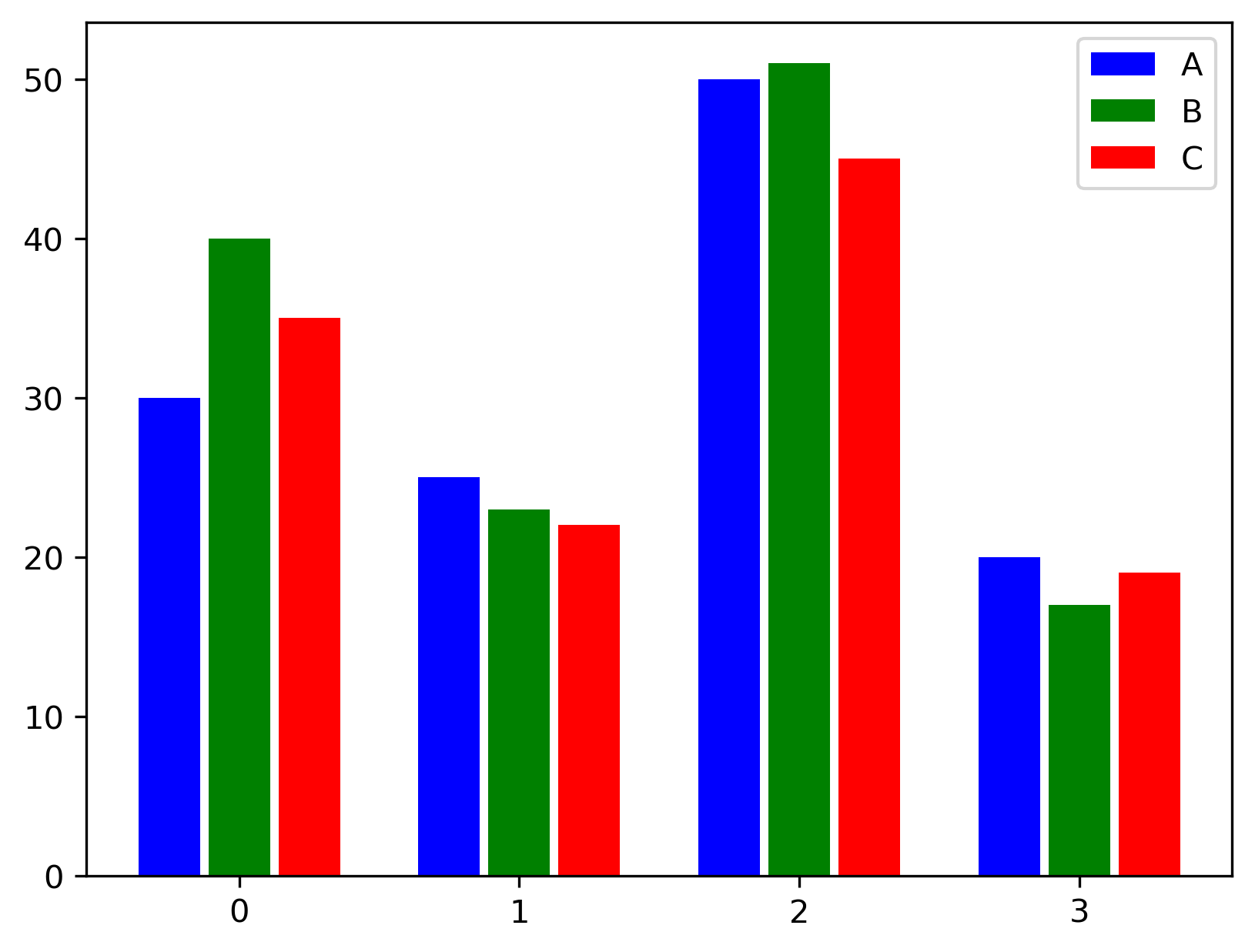### Stacked bars, annotations, and arrow

opacity=0.5
N = 5
menMeans = (20, 35, 30, 35, 27)
womenMeans = (25, 32, 34, 20, 25)
ind = np.arange(N) # the x locations for the groups
bar_width = 0.25

fig, ax = plt.subplots()
ax.bar(ind, menMeans, bar_width, color='r', alpha=opacity)
ax.bar(ind, womenMeans, bar_width, bottom=menMeans, color='b', alpha=opacity)
ax.set_ylabel('Scores')
ax.set_title('Here goes the title')
ax.set_yticks(np.arange(0, 81, 10))
ax.legend(labels=['Men', 'Women'])
ax.grid(color='gray', alpha=opacity, linestyle='dashed')

## Text and arrow on a plot
plt.text(1.5, 65, 'My custom\n text here', size=8)
plt.arrow(1.5, 65, -1.5, -40, shape='full', lw=2)

plt.xticks(ind,('G1', 'G2', 'G3', 'G4', 'G5'))

plt.savefig('stacked_bar.png',dpi=300,bbox_inches='tight')
plt.close('all')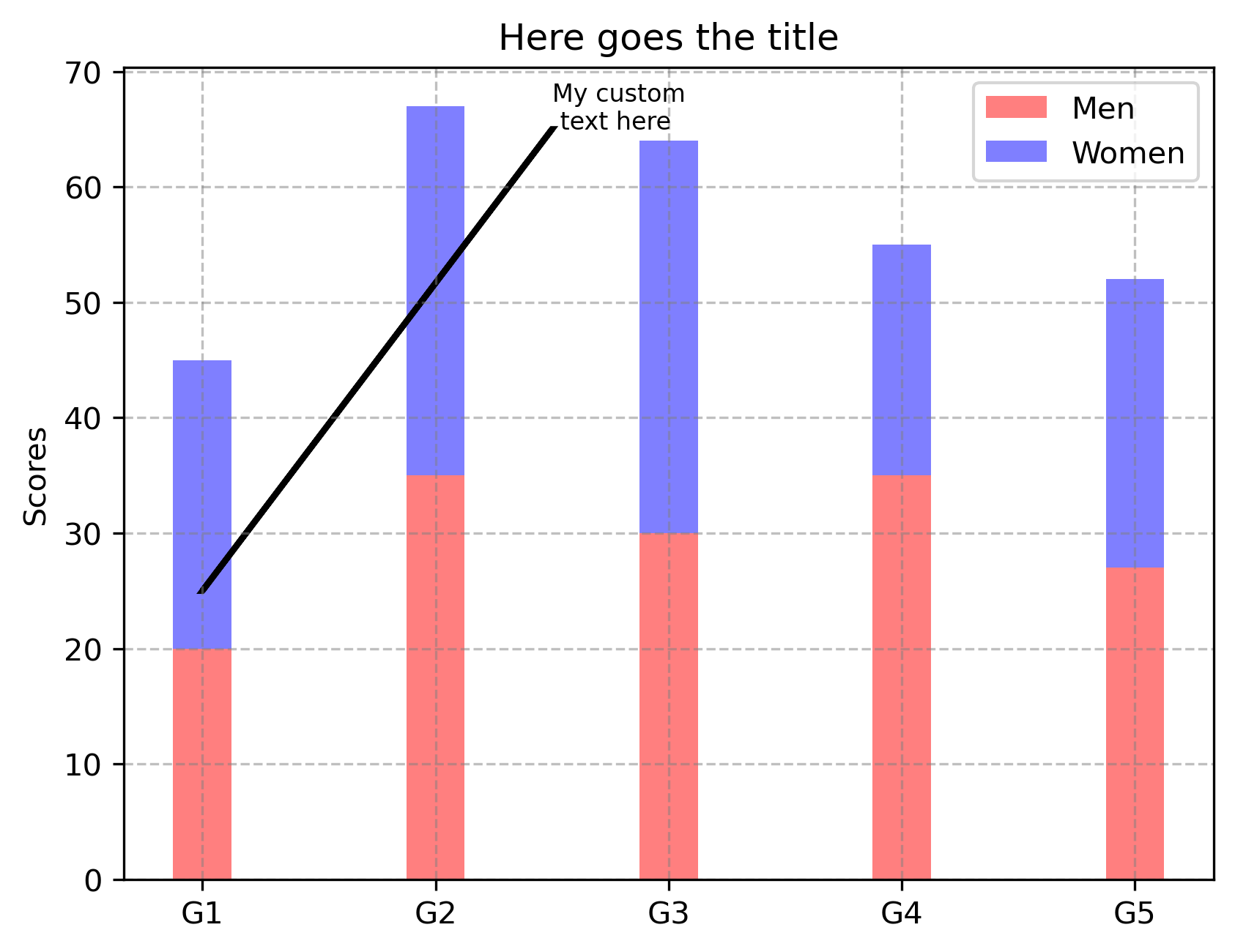## Multiple plots in a figure

import numpy as np
import matplotlib.pyplot as plt

plt.style.use('seaborn')

# make fake data
x_orig = np.linspace(0, 4, 50) # points between 0 and 4

y_orig = np.exp(-x_orig) + 0.01*np.random.normal(size=len(x_orig)) # data is theory plus noise
y_orig2 = np.exp(-x_orig) + 0.05*np.random.normal(size=len(x_orig))
y_orig3 = np.exp(-x_orig) + 0.1*np.random.normal(size=len(x_orig))

fig, (ax1, ax2, ax3) = plt.subplots(3,1,figsize=(10,6),sharex=True)
ax1.plot(x_orig, y_orig, 'b', label='less noise', lw=1) # points
ax1.legend()

ax2.plot(x_orig, y_orig2, 'g', label='more noise', lw=1) # points
ax2.legend()
ax2.set_ylabel("Ylabel")

ax3.plot(x_orig, y_orig3, 'r', label='most noise', lw=1) # points
ax3.legend()
ax3.set_xlabel('Xlabel')

plt.savefig('multiple_plots.png',dpi=300,bbox_inches='tight')
plt.close('all')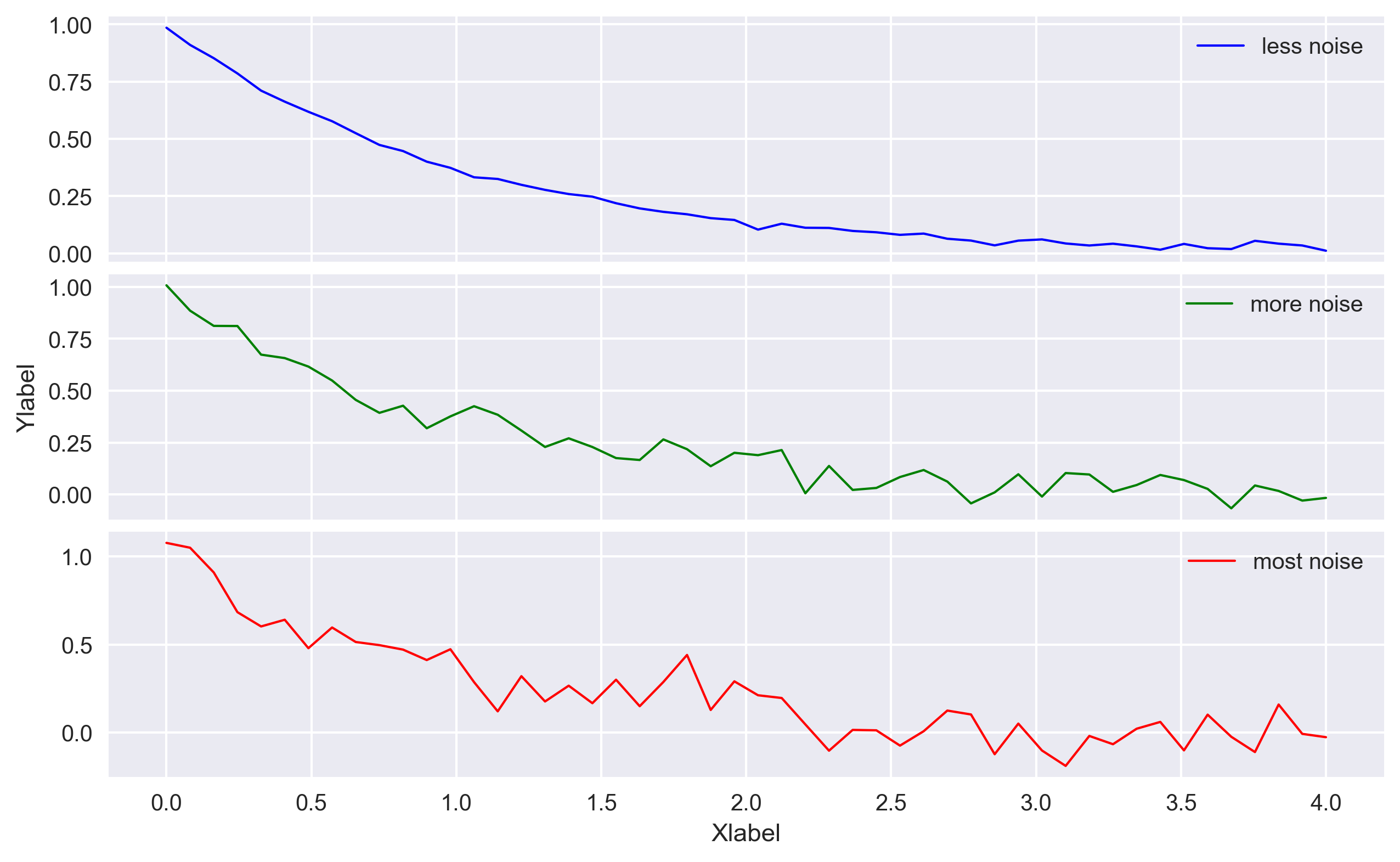## Plotting histograms

I like to plot histograms with the help of the pandas library as it provides a neat plot and offers several methods to manipulate and analyze the data. It is one of the most common way to visualize the distribution of continuous data over an interval (bin). Each bar in a histogram represents the tabulated frequency at each interval.

Histograms also give a rough view of the probability distribution of the data.

import numpy as np
import matplotlib.pyplot as plt
import pandas as pd

means = 20, 50
stdevs = 4, 2
dist = pd.DataFrame(np.random.normal(loc=means, scale=stdevs, size=(1000, 2)),columns=['a', 'b'])

opacity = 0.5
bin_width = 0.8

fig, ax = plt.subplots()
# n, bins, patches = ax.hist(x=dist['a'], bins='auto', color='#0504aa',alpha=opacity, rwidth=bin_width)

dist.plot.kde(ax=ax, legend=False, title='My histogram', color=['r','b'])
dist.plot.hist(density=True,bins=22, alpha=opacity, ax=ax, backend='matplotlib', grid=True, color=['r','b'])
plt.xlabel('Value')
plt.ylabel('Frequency')

plt.savefig('histograms.png',dpi=300,bbox_inches='tight')
plt.close('all')